# DES and 3DES Algorithms¶

DES (Data Encryption Algorithm) is to encipher and decipher 64 bit data blocks using 64 bit key. The key is scheduled to construct 16 round keys. These round keys are used in encryption and decryption flow.

In DES specification, data blocks and keys are composed of bits numbered from left to right, meaning that the left most bit is bit one.

3DES (Triple DES) is an enhancement of DES. It needs 3 keys, and consists of 3 rounds of DES.

## Algorithm Flow¶

The encryption flow is shown in the following figure. It consists initial permutation, 16 rounds of process using round keys and final permutation.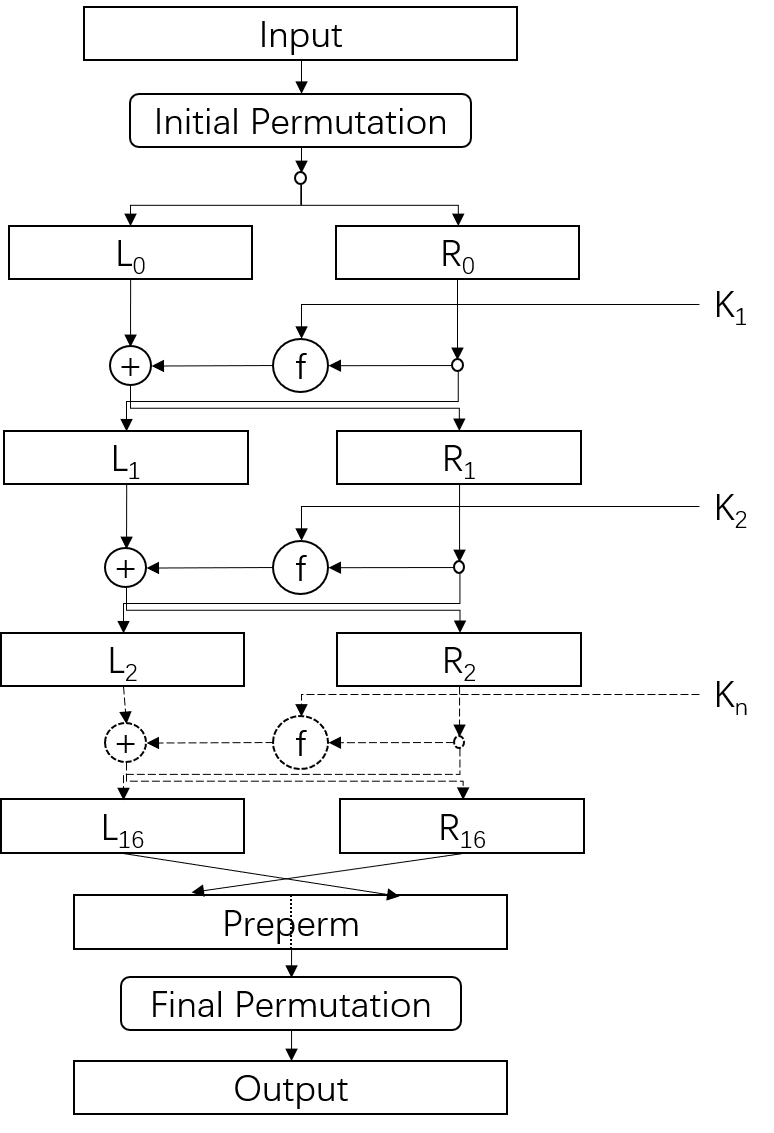The decryption uses the similar flow in encryption, except the round keys are in reversed order.

The function f used in encryption and decryption is depicted in the following figure.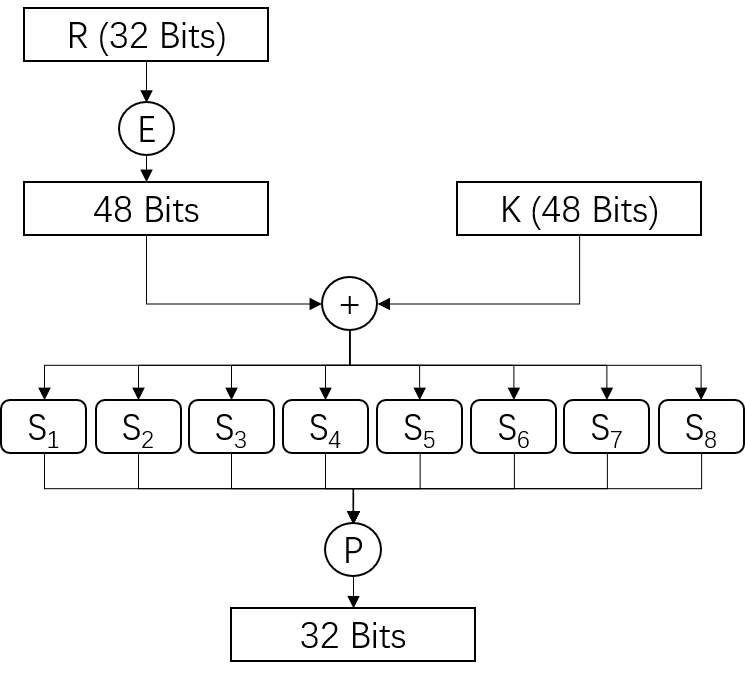Key schedule generates 16 round keys from original key. The process consists of an initial permuted choice and 16 rounds of left shifting and permuted choice.

The 3DES encryption flow is shown in the following figure.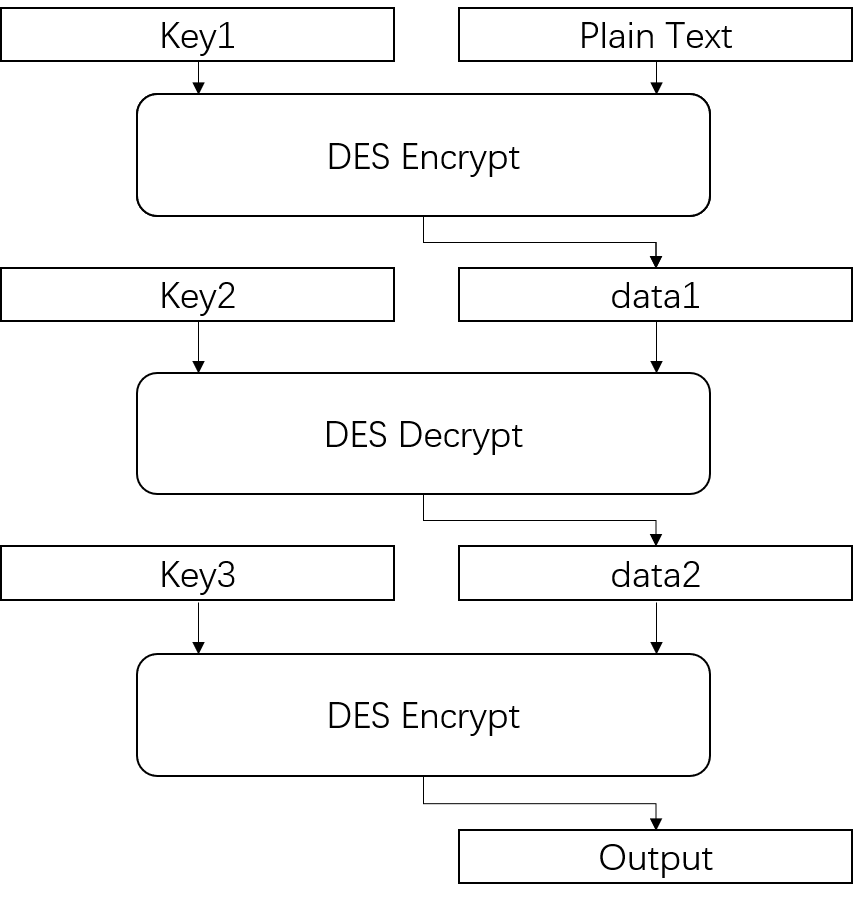The 3DES decryption flow is shown in the following figure.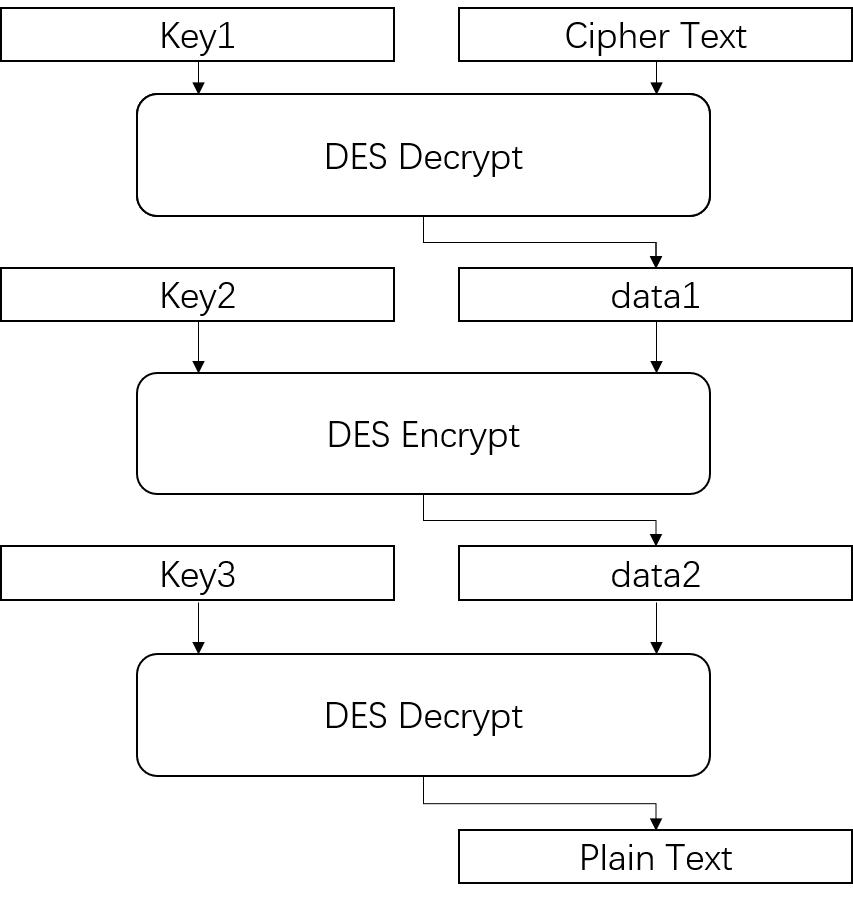## Optimized Implementation on FPGA¶

Key schedule just contains permuted choice and left shifting, which generates a mapping from bit positions in original key and bit positions in each round key. As a result, we can calculate the mapping beforehand and make the entire process in key schedule into direct assignments of round keys.

The data block and key are using different endian approach from arbitrary precision data type defined in HLS, so endian convertion for original data block and key is added before and after encryption and decryption.

The implemented DES encryption/decryption flow is shown in the following figure.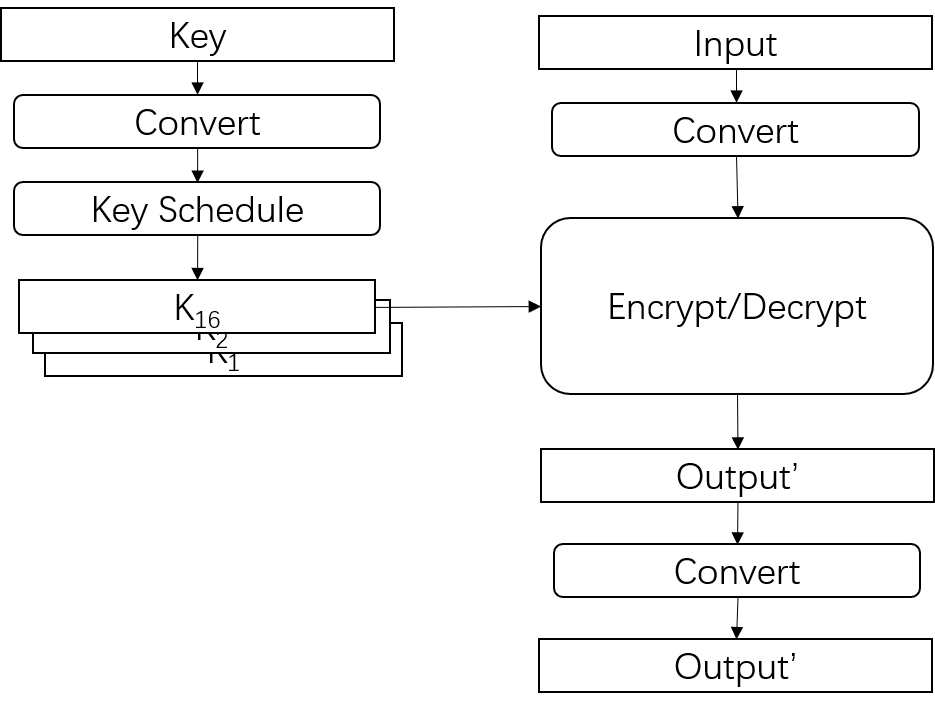The implemented 3DES flow uses dataflow among three DES modules.

## Performance (Device: VU9P)¶

### DES encryption¶

II CLB LUT FF DSP BRAM SRL URAM CP(ns)
1 363 1870 2940 0 0 352 0 1.314

### DES decryption¶

II CLB LUT FF DSP BRAM SRL URAM CP(ns)
1 363 1857 2940 0 0 352 0 1.408

### 3DES encryption¶

II CLB LUT FF DSP BRAM SRL URAM CP(ns)
1 1176 5770 9175 0 0 1224 0 1.510

### 3DES decryption¶

II CLB LUT FF DSP BRAM SRL URAM CP(ns)
1 1141 5734 9175 0 0 1224 0 1.544